## C# || How To Get The Number Of Binary Tree Paths Equal To Path Sum Using C#The following is a module with functions which demonstrates how to get the number of binary tree paths equal to path sum using C#.

1. Number Of Path Sum Paths – Problem Statement

Given the root of a binary tree and an integer targetSum, return the number of paths where the sum of the values along the path equals targetSum.

The path does not need to start or end at the root or a leaf, but it must go downwards (i.e., traveling only from parent nodes to child nodes).

Example 1: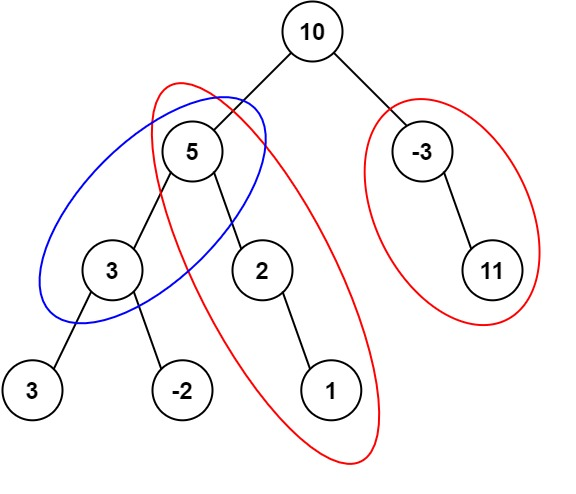``` Input: root = [10,5,-3,3,2,null,11,3,-2,null,1], targetSum = 8 Output: 3 Explanation: The paths that sum to 8 are shown. ```

Example 2:

``` Input: root = [5,4,8,11,null,13,4,7,2,null,null,5,1], targetSum = 22 Output: 3 ```

2. Number Of Path Sum Paths – Solution

The following is a solution which demonstrates how to get the number of binary tree paths equal to path sum.

The main idea here is that the sum at each level for each path is calculated. When the next level is explored, the value at the previous level is summed together with the node value at the current level.

A map dictionary is used to keep track of the sum at each level. If the prefix sum at the previous level is enough to equal the target path sum at the current level, the result count is incremented.

``` 2. Number Of Path Sum Paths - Solution C# // ============================================================================ // Author: Kenneth Perkins // Date: Oct 18, 2021 // Taken From: http://programmingnotes.org/ // File: Solution.cs // Description: Determines how to get the number of tree paths equal to path sum // ============================================================================ /** * Definition for a binary tree node. * public class TreeNode { * public int val; * public TreeNode left; * public TreeNode right; * public TreeNode(int val=0, TreeNode left=null, TreeNode right=null) { * this.val = val; * this.left = left; * this.right = right; * } * } */ public class Solution { private int result = 0; private Dictionary<int, int> sumFrequency = new Dictionary<int, int>(); public int PathSum(TreeNode root, int targetSum) { sumFrequency = 1; Search(root, targetSum, 0); return result; } public void Search(TreeNode node, int targetSum, int currentSum) { if (node == null) { return; } // Determine the current sum currentSum = currentSum + node.val; // Get the path prefix sum var prefixSum = currentSum - targetSum; if (sumFrequency.ContainsKey(prefixSum)) { result += sumFrequency[prefixSum]; } // Increment the number of times this prefix has been seen sumFrequency[currentSum] = (sumFrequency.ContainsKey(currentSum) ? sumFrequency[currentSum] : 0) + 1; // Keep exploring along branches finding the target sum Search(node.left, targetSum, currentSum); Search(node.right, targetSum, currentSum); // Remove value of this prefixSum (path's been explored) --sumFrequency[currentSum]; } }// http://programmingnotes.org/ 1234567891011121314151617181920212223242526272829303132333435363738394041424344454647484950515253545556 // ============================================================================//    Author: Kenneth Perkins//    Date:   Oct 18, 2021//    Taken From: http://programmingnotes.org///    File:  Solution.cs//    Description: Determines how to get the number of tree paths equal to path sum// ============================================================================/** * Definition for a binary tree node. * public class TreeNode { *     public int val; *     public TreeNode left; *     public TreeNode right; *     public TreeNode(int val=0, TreeNode left=null, TreeNode right=null) { *         this.val = val; *         this.left = left; *         this.right = right; *     } * } */public class Solution {    private int result = 0;    private Dictionary<int, int> sumFrequency = new Dictionary<int, int>();     public int PathSum(TreeNode root, int targetSum) {        sumFrequency = 1;        Search(root, targetSum, 0);        return result;    }     public void Search(TreeNode node, int targetSum, int currentSum) {        if (node == null) {            return;        }         // Determine the current sum        currentSum = currentSum + node.val;         // Get the path prefix sum        var prefixSum = currentSum - targetSum;         if (sumFrequency.ContainsKey(prefixSum)) {            result += sumFrequency[prefixSum];        }         // Increment the number of times this prefix has been seen        sumFrequency[currentSum] = (sumFrequency.ContainsKey(currentSum) ? sumFrequency[currentSum] : 0) + 1;         // Keep exploring along branches finding the target sum        Search(node.left, targetSum, currentSum);        Search(node.right, targetSum, currentSum);         // Remove value of this prefixSum (path's been explored)        --sumFrequency[currentSum];    }}// http://programmingnotes.org/ ```

QUICK NOTES:
The highlighted lines are sections of interest to look out for.

The code is heavily commented, so no further insight is necessary. If you have any questions, feel free to leave a comment below.

Once compiled, you should get this as your output for the example cases:

``` 3 3 ```

## C# || How To Get All Root To Leaf Binary Tree Paths Equal To Path Sum Using C#The following is a module with functions which demonstrates how to get all the root to leaf binary tree paths equal to path sum using C#.

1. Root To Leaf Path Sum – Problem Statement

Given the root of a binary tree and an integer targetSum, return all root-to-leaf paths where the sum of the node values in the path equals targetSum. Each path should be returned as a list of the node values, not node references.

A root-to-leaf path is a path starting from the root and ending at any leaf node. A leaf is a node with no children.

Example 1: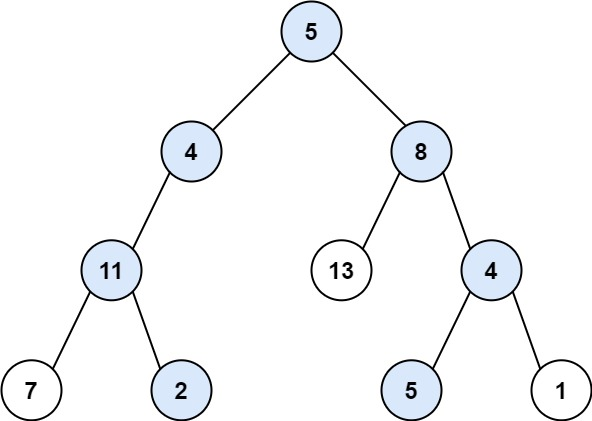``` Input: root = [5,4,8,11,null,13,4,7,2,null,null,5,1], targetSum = 22 Output: [[5,4,11,2],[5,8,4,5]] Explanation: There are two paths whose sum equals targetSum: 5 + 4 + 11 + 2 = 22 5 + 8 + 4 + 5 = 22 ```

Example 2: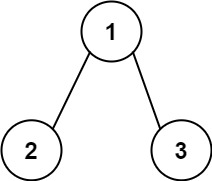``` Input: root = [1,2,3], targetSum = 5 Output: [] ```

Example 3:

``` Input: root = [1,2], targetSum = 0 Output: [] ```

2. Root To Leaf Path Sum – Solution

The following is a solution which demonstrates how to get all the root to leaf binary tree paths equal to path sum.

The main idea here is that the sum at each level for each path is calculated until we reach the end of the root-to-leaf.

A list is used to store the node value at each level. When the next level is explored, the value is appended to the list, and the process continues.

When we reach the end of the leaf, we check to see if the target value has been reached. If so, we add the node values that make up the path to the result list.

``` 2. Root To Leaf Path Sum - Solution C# // ============================================================================ // Author: Kenneth Perkins // Date: Oct 18, 2021 // Taken From: http://programmingnotes.org/ // File: Solution.cs // Description: Determines how to get all root to leaf path sum in a tree // ============================================================================ /** * Definition for a binary tree node. * public class TreeNode { * public int val; * public TreeNode left; * public TreeNode right; * public TreeNode(int val=0, TreeNode left=null, TreeNode right=null) { * this.val = val; * this.left = left; * this.right = right; * } * } */ public class Solution { private List<IList<int>> result = new List<IList<int>>(); public IList<IList<int>> PathSum(TreeNode root, int targetSum) { Search(root, targetSum, 0, new List<int>()); return result; } private void Search(TreeNode node, int targetSum, int currentSum, List<int> path) { if (node == null) { return; } // Add the node to this path path.Add(node.val); // Add the current value to the running total currentSum = currentSum + node.val; // Since this is a root-to-leaf check, evaluate for the // success condition when both left and right nodes are null if (node.left == null && node.right == null) { // Check to see if current value equals target if (currentSum == targetSum) { result.Add(new List<int>(path)); } } // Keep exploring along branches finding the target sum Search(node.left, targetSum, currentSum, path); Search(node.right, targetSum, currentSum, path); // Remove the last item added as this path has already been explored path.RemoveAt(path.Count - 1); } }// http://programmingnotes.org/ 123456789101112131415161718192021222324252627282930313233343536373839404142434445464748495051525354555657 // ============================================================================//    Author: Kenneth Perkins//    Date:   Oct 18, 2021//    Taken From: http://programmingnotes.org///    File:  Solution.cs//    Description: Determines how to get all root to leaf path sum in a tree// ============================================================================/** * Definition for a binary tree node. * public class TreeNode { *     public int val; *     public TreeNode left; *     public TreeNode right; *     public TreeNode(int val=0, TreeNode left=null, TreeNode right=null) { *         this.val = val; *         this.left = left; *         this.right = right; *     } * } */public class Solution {    private List<IList<int>> result = new List<IList<int>>();     public IList<IList<int>> PathSum(TreeNode root, int targetSum) {        Search(root, targetSum, 0, new List<int>());        return result;    }     private void Search(TreeNode node, int targetSum, int currentSum, List<int> path) {        if (node == null) {            return;        }         // Add the node to this path        path.Add(node.val);         // Add the current value to the running total        currentSum = currentSum + node.val;         // Since this is a root-to-leaf check, evaluate for the        // success condition when both left and right nodes are null        if (node.left == null && node.right == null) {             // Check to see if current value equals target            if (currentSum == targetSum) {                result.Add(new List<int>(path));            }        }         // Keep exploring along branches finding the target sum        Search(node.left, targetSum, currentSum, path);        Search(node.right, targetSum, currentSum, path);         // Remove the last item added as this path has already been explored        path.RemoveAt(path.Count - 1);    }}// http://programmingnotes.org/ ```

QUICK NOTES:
The highlighted lines are sections of interest to look out for.

The code is heavily commented, so no further insight is necessary. If you have any questions, feel free to leave a comment below.

Once compiled, you should get this as your output for the example cases:

``` [[5,4,11,2],[5,8,4,5]] [] [] ```

## C# || How To Determine If Binary Tree Root To Leaf Path Sum Exists Using C#The following is a module with functions which demonstrates how to determine if a binary tree root to leaf path sum exists using C#.

1. Has Path Sum – Problem Statement

Given the root of a binary tree and an integer targetSum, return true if the tree has a root-to-leaf path such that adding up all the values along the path equals targetSum.

A leaf is a node with no children.

Example 1: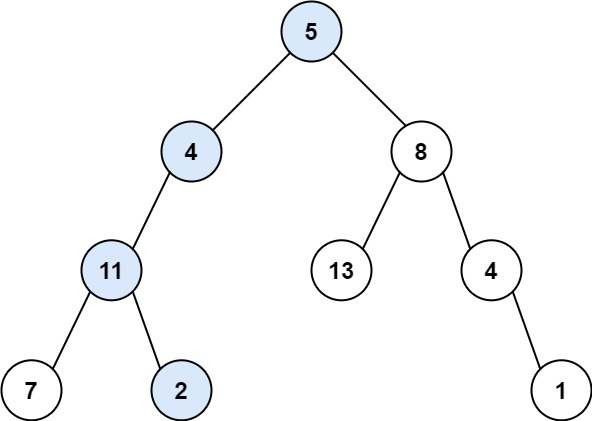``` Input: root = [5,4,8,11,null,13,4,7,2,null,null,null,1], targetSum = 22 Output: true ```

Example 2:``` Input: root = [1,2,3], targetSum = 5 Output: false ```

Example 3:

``` Input: root = [1,2], targetSum = 0 Output: false ```

2. Has Path Sum – Solution

The following is a solution which demonstrates how to determine if a binary tree root to leaf path sum exists.

``` 2. Has Path Sum - Solution C# // ============================================================================ // Author: Kenneth Perkins // Date: Oct 18, 2021 // Taken From: http://programmingnotes.org/ // File: Solution.cs // Description: Determines if a root to leaf path sum exists in a binary tree // ============================================================================ /** * Definition for a binary tree node. * public class TreeNode { * public int val; * public TreeNode left; * public TreeNode right; * public TreeNode(int val=0, TreeNode left=null, TreeNode right=null) { * this.val = val; * this.left = left; * this.right = right; * } * } */ public class Solution { public bool HasPathSum(TreeNode root, int targetSum) { return Search(root, targetSum); } public bool Search(TreeNode node, int targetSum) { if (node == null) { return false; } // Determine the current sum targetSum = targetSum - node.val; // Since this is a root-to-leaf check, evaluate for the // success condition when both left and right nodes are null if (node.left == null && node.right == null) { return targetSum == 0; } // Keep exploring along branches finding the target sum return Search(node.left, targetSum) || Search(node.right, targetSum); } }// http://programmingnotes.org/ 1234567891011121314151617181920212223242526272829303132333435363738394041424344 // ============================================================================//    Author: Kenneth Perkins//    Date:   Oct 18, 2021//    Taken From: http://programmingnotes.org///    File:  Solution.cs//    Description: Determines if a root to leaf path sum exists in a binary tree// ============================================================================/** * Definition for a binary tree node. * public class TreeNode { *     public int val; *     public TreeNode left; *     public TreeNode right; *     public TreeNode(int val=0, TreeNode left=null, TreeNode right=null) { *         this.val = val; *         this.left = left; *         this.right = right; *     } * } */public class Solution {    public bool HasPathSum(TreeNode root, int targetSum) {        return Search(root, targetSum);    }     public bool Search(TreeNode node, int targetSum) {        if (node == null) {            return false;        }         // Determine the current sum        targetSum = targetSum - node.val;         // Since this is a root-to-leaf check, evaluate for the        // success condition when both left and right nodes are null        if (node.left == null && node.right == null) {            return targetSum == 0;        }         // Keep exploring along branches finding the target sum        return Search(node.left, targetSum)            || Search(node.right, targetSum);    }}// http://programmingnotes.org/ ```

QUICK NOTES:
The highlighted lines are sections of interest to look out for.

The code is heavily commented, so no further insight is necessary. If you have any questions, feel free to leave a comment below.

Once compiled, you should get this as your output for the example cases:

``` true false false ```

## C# || How To Convert Sorted Array to Binary Search Tree Using C#The following is a module with functions which demonstrates how to convert a sorted array to a binary search tree using C#.

1. Sorted Array To BST – Problem Statement

Given an integer array nums where the elements are sorted in ascending order, convert it to a height-balanced binary search tree.

A height-balanced binary tree is a binary tree in which the depth of the two subtrees of every node never differs by more than one.

Example 1: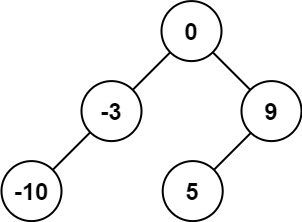``` Input: nums = [-10,-3,0,5,9] Output: [0,-3,9,-10,null,5] Explanation: [0,-10,5,null,-3,null,9] is also accepted: ```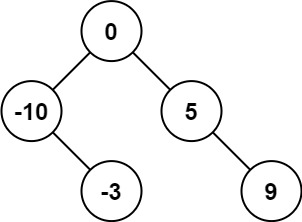Example 2: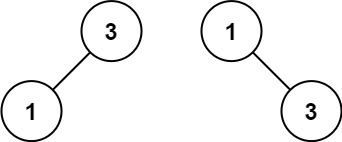``` Input: nums = [1,3] Output: [3,1] Explanation: [1,3] and [3,1] are both a height-balanced BSTs. ```

2. Sorted Array To BST – Solution

The following is a solution which demonstrates how to convert a sorted array to a binary search tree.

``` 2. Sorted Array To BST - Solution C# // ============================================================================ // Author: Kenneth Perkins // Date: Oct 18, 2021 // Taken From: http://programmingnotes.org/ // File: Solution.cs // Description: Demonstrates how to convert sorted array to BST // ============================================================================ /** * Definition for a binary tree node. * public class TreeNode { * public int val; * public TreeNode left; * public TreeNode right; * public TreeNode(int val=0, TreeNode left=null, TreeNode right=null) { * this.val = val; * this.left = left; * this.right = right; * } * } */ public class Solution { public TreeNode SortedArrayToBST(int[] nums) { return BuildBST(nums, 0, nums.Length -1); } TreeNode BuildBST(int[] nums, int start, int end) { if (start > end) { return null; } var mid = start + (end - start) / 2; var node = new TreeNode(nums[mid]); node.left = BuildBST(nums, start, mid - 1); node.right = BuildBST(nums, mid + 1, end); return node; } }// http://programmingnotes.org/ 12345678910111213141516171819202122232425262728293031323334353637 // ============================================================================//    Author: Kenneth Perkins//    Date:   Oct 18, 2021//    Taken From: http://programmingnotes.org///    File:  Solution.cs//    Description: Demonstrates how to convert sorted array to BST// ============================================================================/** * Definition for a binary tree node. * public class TreeNode { *     public int val; *     public TreeNode left; *     public TreeNode right; *     public TreeNode(int val=0, TreeNode left=null, TreeNode right=null) { *         this.val = val; *         this.left = left; *         this.right = right; *     } * } */public class Solution {    public TreeNode SortedArrayToBST(int[] nums) {        return BuildBST(nums, 0, nums.Length -1);    }     TreeNode BuildBST(int[] nums, int start, int end) {        if (start > end) {            return null;        }         var mid = start + (end - start) / 2;        var node = new TreeNode(nums[mid]);        node.left = BuildBST(nums, start, mid - 1);        node.right = BuildBST(nums, mid + 1, end);        return node;    }}// http://programmingnotes.org/ ```

QUICK NOTES:
The highlighted lines are sections of interest to look out for.

The code is heavily commented, so no further insight is necessary. If you have any questions, feel free to leave a comment below.

Once compiled, you should get this as your output for the example cases:

``` [0,-10,5,null,-3,null,9] [1,null,3] ```

## C# || How To Convert 1D Array Into 2D Array Using C#The following is a module with functions which demonstrates how to convert a 1D array into a 2D array using C#.

1. Construct 2D Array – Problem Statement

You are given a 0-indexed 1-dimensional (1D) integer array original, and two integers, m and n. You are tasked with creating a 2-dimensional (2D) array with m rows and n columns using all the elements from original.

The elements from indices 0 to n – 1 (inclusive) of original should form the first row of the constructed 2D array, the elements from indices n to 2 * n – 1 (inclusive) should form the second row of the constructed 2D array, and so on.

Return an m x n 2D array constructed according to the above procedure, or an empty 2D array if it is impossible.

Example 1: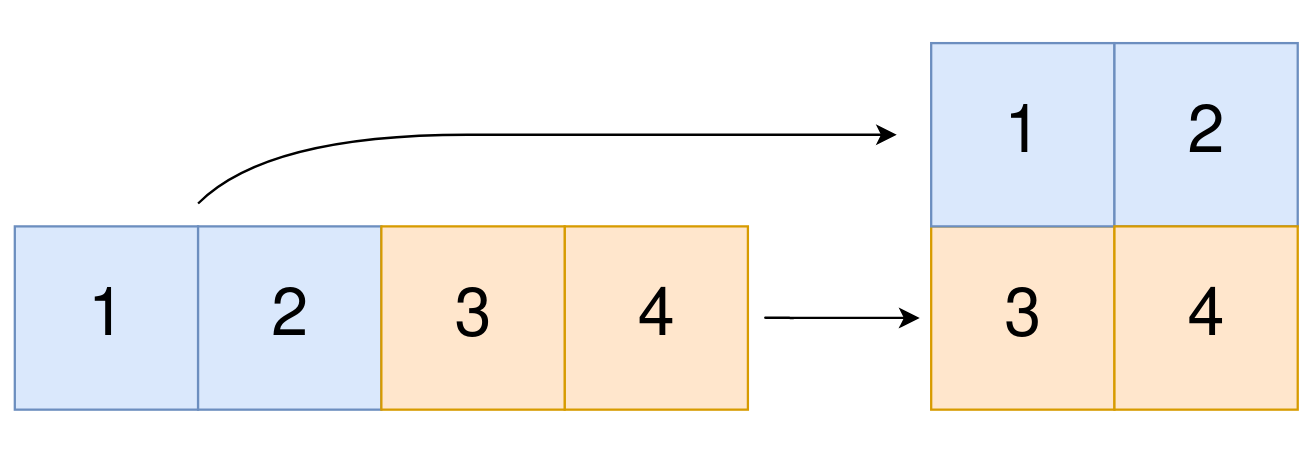``` Input: original = [1,2,3,4], m = 2, n = 2 Output: [[1,2],[3,4]] Explanation: The constructed 2D array should contain 2 rows and 2 columns. The first group of n=2 elements in original, [1,2], becomes the first row in the constructed 2D array. The second group of n=2 elements in original, [3,4], becomes the second row in the constructed 2D array. ```

Example 2:

``` Input: original = [1,2,3], m = 1, n = 3 Output: [[1,2,3]] Explanation: The constructed 2D array should contain 1 row and 3 columns. Put all three elements in original into the first row of the constructed 2D array. ```

Example 3:

``` Input: original = [1,2], m = 1, n = 1 Output: [] Explanation: There are 2 elements in original. It is impossible to fit 2 elements in a 1x1 2D array, so return an empty 2D array. ```

Example 4:

``` Input: original = , m = 1, n = 2 Output: [] Explanation: There is 1 element in original. It is impossible to make 1 element fill all the spots in a 1x2 2D array, so return an empty 2D array. ```

2. Construct 2D Array – Solution

The following is a solution which demonstrates how to convert a 1D array into a 2D array.

``` 2. Construct 2D Array - Solution C# // ============================================================================ // Author: Kenneth Perkins // Date: Oct 18, 2021 // Taken From: http://programmingnotes.org/ // File: Solution.cs // Description: Demonstrates how to convert a 1D array into a 2D array // ============================================================================ public class Solution { public int[][] Construct2DArray(int[] original, int m, int n) { // Determine if parameters are valid if (original.Length != m * n) { return new int[]; } // Create 2D array var result = new int[m][]; for (int row = 0; row < result.Length; ++row) { result[row] = new int[n]; } // Populate result for (int index = 0; index < original.Length; ++index) { // Determine the result array row and column index var rowIndex = index / n; var colIndex = index % n; // Copy values to result result[rowIndex][colIndex] = original[index]; } return result; } }// http://programmingnotes.org/ 123456789101112131415161718192021222324252627282930313233 // ============================================================================//    Author: Kenneth Perkins//    Date:   Oct 18, 2021//    Taken From: http://programmingnotes.org///    File:  Solution.cs//    Description: Demonstrates how to convert a 1D array into a 2D array// ============================================================================public class Solution {    public int[][] Construct2DArray(int[] original, int m, int n) {        // Determine if parameters are valid        if (original.Length != m * n) {            return new int[];        }         // Create 2D array        var result = new int[m][];        for (int row = 0; row < result.Length; ++row) {            result[row] = new int[n];        }         // Populate result        for (int index = 0; index < original.Length; ++index) {            // Determine the result array row and column index            var rowIndex = index / n;            var colIndex = index % n;             // Copy values to result            result[rowIndex][colIndex] = original[index];        }         return result;    }}// http://programmingnotes.org/ ```

QUICK NOTES:
The highlighted lines are sections of interest to look out for.

The code is heavily commented, so no further insight is necessary. If you have any questions, feel free to leave a comment below.

Once compiled, you should get this as your output for the example cases:

``` [[1,2],[3,4]] [[1,2,3]] [] [] ```

## C# || How To Find Cousins In A Binary Tree Using C#The following is a module with functions which demonstrates how to find the cousins in a binary tree using C#.

1. Is Cousins – Problem Statement

Given the root of a binary tree with unique values and the values of two different nodes of the tree x and y, return true if the nodes corresponding to the values x and y in the tree are cousins, or false otherwise.

Two nodes of a binary tree are cousins if they have the same depth with different parents.

Note that in a binary tree, the root node is at the depth 0, and children of each depth k node are at the depth k + 1.

Example 1: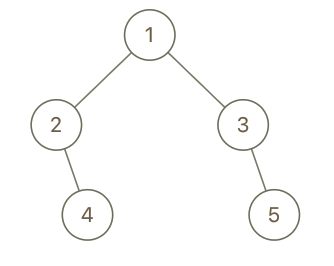``` Input: root = [1,2,3,4], x = 4, y = 3 Output: false ```

Example 2:``` Input: root = [1,2,3,null,4,null,5], x = 5, y = 4 Output: true ```

Example 3:``` Input: root = [1,2,3,null,4], x = 2, y = 3 Output: false ```

2. Is Cousins – Solution

The following is a solution which demonstrates how to determine if x and y values are cousins in a binary tree.

The idea here is to explore each path, finding the node values that represent x and y.

Once both values are found, save the depth and parent node, and determine if they are cousins

``` 2. Is Cousins - Solution C# // ============================================================================ // Author: Kenneth Perkins // Date: Oct 17, 2021 // Taken From: http://programmingnotes.org/ // File: Solution.cs // Description: Determines if x and y are cousins in a binary tree // ============================================================================ /** * Definition for a binary tree node. * public class TreeNode { * public int val; * public TreeNode left; * public TreeNode right; * public TreeNode(int val=0, TreeNode left=null, TreeNode right=null) { * this.val = val; * this.left = left; * this.right = right; * } * } */ public class Solution { // For the x parent and depth values private int xParent = -1; private int xDepth = -1; // For the y parent and depth values private int yParent = -1; private int yDepth = -1; public bool IsCousins(TreeNode root, int x, int y) { Search(root, x, y, root.val, 0); // Check for success condition return xParent != yParent && xDepth == yDepth; } private void Search(TreeNode node, int x, int y, int parent, int depth) { // Values have been found if (xParent != -1 && yParent != -1) { return; } if (node == null) { return; } if (node.val == x) { // Set the x parent and depth values xParent = parent; xDepth = depth; } else if (node.val == y) { // Set the y parent and depth values yParent = parent; yDepth = depth; } else { // Keep exploring along branches for the success condition Search(node.left, x, y, node.val, depth + 1); Search(node.right, x, y, node.val, depth + 1); } } }// http://programmingnotes.org/ 12345678910111213141516171819202122232425262728293031323334353637383940414243444546474849505152535455565758596061 // ============================================================================//    Author: Kenneth Perkins//    Date:   Oct 17, 2021//    Taken From: http://programmingnotes.org///    File:  Solution.cs//    Description: Determines if x and y are cousins in a binary tree// ============================================================================/** * Definition for a binary tree node. * public class TreeNode { *     public int val; *     public TreeNode left; *     public TreeNode right; *     public TreeNode(int val=0, TreeNode left=null, TreeNode right=null) { *         this.val = val; *         this.left = left; *         this.right = right; *     } * } */public class Solution {    // For the x parent and depth values    private int xParent = -1;    private int xDepth = -1;     // For the y parent and depth values    private int yParent = -1;    private int yDepth = -1;     public bool IsCousins(TreeNode root, int x, int y) {        Search(root, x, y, root.val, 0);         // Check for success condition        return xParent != yParent && xDepth == yDepth;    }     private void Search(TreeNode node, int x, int y, int parent, int depth) {        // Values have been found        if (xParent != -1 && yParent != -1) {            return;        }         if (node == null) {            return;        }         if (node.val == x) {            // Set the x parent and depth values            xParent = parent;            xDepth = depth;        } else if (node.val == y) {            // Set the y parent and depth values            yParent = parent;            yDepth = depth;        } else {            // Keep exploring along branches for the success condition            Search(node.left, x, y, node.val, depth + 1);            Search(node.right, x, y, node.val, depth + 1);        }    }}// http://programmingnotes.org/ ```

QUICK NOTES:
The highlighted lines are sections of interest to look out for.

The code is heavily commented, so no further insight is necessary. If you have any questions, feel free to leave a comment below.

Once compiled, you should get this as your output for the example cases:

``` false true false ```

## C# || How To Construct A Binary Search Tree From Preorder Traversal Using C#The following is a module with functions which demonstrates how to construct a binary search tree from preorder traversal using C#.

1. Binary Tree From Preorder – Problem Statement

Given an array of integers preorder, which represents the preorder traversal of a BST (i.e., binary search tree), construct the tree and return its root.

It is guaranteed that there is always possible to find a binary search tree with the given requirements for the given test cases.

A binary search tree is a binary tree where for every node, any descendant of Node.left has a value strictly less than Node.val, and any descendant of Node.right has a value strictly greater than Node.val.

A preorder traversal of a binary tree displays the value of the node first, then traverses Node.left, then traverses Node.right.

Example 1: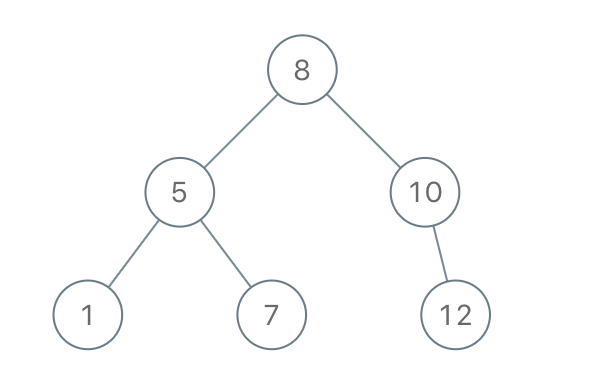``` Input: preorder = [8,5,1,7,10,12] Output: [8,5,10,1,7,null,12] ```

Example 2:

``` Input: preorder = [1,3] Output: [1,null,3] ```

2. Binary Tree From Preorder – Solution

The following is a solution which demonstrates how to construct a binary search tree from preorder traversal.

``` 2. Binary Tree From Preorder - Solution C# // ============================================================================ // Author: Kenneth Perkins // Date: Oct 16, 2021 // Taken From: http://programmingnotes.org/ // File: Solution.cs // Description: Demonstrates how to construct a BST from preorder traversal // ============================================================================ /** * Definition for a binary tree node. * public class TreeNode { * public int val; * public TreeNode left; * public TreeNode right; * public TreeNode(int val=0, TreeNode left=null, TreeNode right=null) { * this.val = val; * this.left = left; * this.right = right; * } * } */ public class Solution { private int currentIndex = 0; public TreeNode BstFromPreorder(int[] preorder) { return BuildBST(preorder, int.MaxValue); } TreeNode BuildBST(int[] preorder, int parentValue) { if (currentIndex == preorder.Length || preorder[currentIndex] > parentValue) { return null; } var currentValue = preorder[currentIndex++]; var node = new TreeNode(currentValue); node.left = BuildBST(preorder, currentValue); node.right = BuildBST(preorder, parentValue); return node; } }// http://programmingnotes.org/ 123456789101112131415161718192021222324252627282930313233343536373839 // ============================================================================//    Author: Kenneth Perkins//    Date:   Oct 16, 2021//    Taken From: http://programmingnotes.org///    File:  Solution.cs//    Description: Demonstrates how to construct a BST from preorder traversal// ============================================================================/** * Definition for a binary tree node. * public class TreeNode { *     public int val; *     public TreeNode left; *     public TreeNode right; *     public TreeNode(int val=0, TreeNode left=null, TreeNode right=null) { *         this.val = val; *         this.left = left; *         this.right = right; *     } * } */public class Solution {    private int currentIndex = 0;     public TreeNode BstFromPreorder(int[] preorder) {        return BuildBST(preorder, int.MaxValue);    }     TreeNode BuildBST(int[] preorder, int parentValue) {        if (currentIndex == preorder.Length || preorder[currentIndex] > parentValue) {            return null;        }         var currentValue = preorder[currentIndex++];        var node = new TreeNode(currentValue);        node.left = BuildBST(preorder, currentValue);        node.right = BuildBST(preorder, parentValue);        return node;    }}// http://programmingnotes.org/ ```

QUICK NOTES:
The highlighted lines are sections of interest to look out for.

The code is heavily commented, so no further insight is necessary. If you have any questions, feel free to leave a comment below.

Once compiled, you should get this as your output for the example cases:

``` [8,5,10,1,7,null,12] [1,null,3] ```

## C# || How To Find The Next Greater Element In A Circular Array Using C#The following is a module with functions which demonstrates how to find the next greater element in a circular array using C#.

1. Circular Next Greater – Problem Statement

Given a circular integer array nums (i.e., the next element of nums[nums.length – 1] is nums), return the next greater number for every element in nums.

The next greater number of a number x is the first greater number to its traversing-order next in the array, which means you could search circularly to find its next greater number. If it doesn’t exist, return -1 for this number.

Example 1:

``` Input: nums = [1,2,1] Output: [2,-1,2] Explanation: The first 1's next greater number is 2; The number 2 can't find next greater number. The second 1's next greater number needs to search circularly, which is also 2. ```

Example 2:

``` Input: nums = [1,2,3,4,3] Output: [2,3,4,-1,4] ```

2. Circular Next Greater – Solution

The following is a solution which demonstrates how to find the next greater element in a circular array.

This solution uses the monotonic stack approach. This solution finds the next greater element for each array value, in the first pass, and then uses a second pass to process any remaining values since the array is circular.

``` 2. Circular Next Greater - Solution C# // ============================================================================ // Author: Kenneth Perkins // Date: Oct 15, 2021 // Taken From: http://programmingnotes.org/ // File: Solution.cs // Description: Demonstrates how to find the next greater element in a // circular array // ============================================================================ public class Solution { public int[] NextGreaterElements(int[] nums) { var result = GetNextGreatest(nums); return result; } public int[] GetNextGreatest(int[] nums) { var result = new int[nums.Length]; var stack = new Stack<int>(); for (int index = 0; index < nums.Length; ++index) { // Default to -1 for this index result[index] = -1; // Fill in values that have a greater element while (stack.Count > 0 && nums[stack.Peek()] < nums[index]) { result[stack.Peek()] = nums[index]; stack.Pop(); } // Add index to stack stack.Push(index); } // Fill in any left over values since this is circular for (int index = 0; index < nums.Length && stack.Count > 0; ++index) { while (stack.Count > 0 && nums[stack.Peek()] < nums[index]) { result[stack.Peek()] = nums[index]; stack.Pop(); } } return result; } }// http://programmingnotes.org/ 12345678910111213141516171819202122232425262728293031323334353637383940414243 // ============================================================================//    Author: Kenneth Perkins//    Date:   Oct 15, 2021//    Taken From: http://programmingnotes.org///    File:  Solution.cs//    Description: Demonstrates how to find the next greater element in a //        circular array// ============================================================================public class Solution {    public int[] NextGreaterElements(int[] nums) {        var result = GetNextGreatest(nums);        return result;    }     public int[] GetNextGreatest(int[] nums) {        var result = new int[nums.Length];        var stack = new Stack<int>();         for (int index = 0; index < nums.Length; ++index) {            // Default to -1 for this index            result[index] = -1;             // Fill in values that have a greater element            while (stack.Count > 0 && nums[stack.Peek()] < nums[index]) {                result[stack.Peek()] = nums[index];                stack.Pop();            }             // Add index to stack            stack.Push(index);        }         // Fill in any left over values since this is circular        for (int index = 0; index < nums.Length && stack.Count > 0; ++index) {            while (stack.Count > 0 && nums[stack.Peek()] < nums[index]) {                result[stack.Peek()] = nums[index];                stack.Pop();            }        }         return result;    }}// http://programmingnotes.org/ ```

QUICK NOTES:
The highlighted lines are sections of interest to look out for.

The code is heavily commented, so no further insight is necessary. If you have any questions, feel free to leave a comment below.

Once compiled, you should get this as your output for the example cases:

``` [2,-1,2] [2,3,4,-1,4] ```

## C# || How To Find The Next Greater Element In An Array Using C#The following is a module with functions which demonstrates how to find the next greater element in an array using C#.

1. Next Greater – Problem Statement

The next greater element of some element x in an array is the first greater element that is to the right of x in the same array.

You are given two distinct 0-indexed integer arrays nums1 and nums2, where nums1 is a subset of nums2.

For each 0 <= i < nums1.length, find the index j such that nums1[i] == nums2[j] and determine the next greater element of nums2[j] in nums2. If there is no next greater element, then the answer for this query is -1.

Return an array ans of length nums1.length such that ans[i] is the next greater element as described above.

Example 1:

``` Input: nums1 = [4,1,2], nums2 = [1,3,4,2] Output: [-1,3,-1] Explanation: The next greater element for each value of nums1 is as follows: - 4 is underlined in nums2 = [1,3,4,2]. There is no next greater element, so the answer is -1. - 1 is underlined in nums2 = [1,3,4,2]. The next greater element is 3. - 2 is underlined in nums2 = [1,3,4,2]. There is no next greater element, so the answer is -1. ```

Example 2:

``` Input: nums1 = [2,4], nums2 = [1,2,3,4] Output: [3,-1] Explanation: The next greater element for each value of nums1 is as follows: - 2 is underlined in nums2 = [1,2,3,4]. The next greater element is 3. - 4 is underlined in nums2 = [1,2,3,4]. There is no next greater element, so the answer is -1. ```

2. Next Greater – Solution

The following is a solution which demonstrates how to find the next greater element in an array.

This solution uses the monotonic stack approach. This solution finds the next greater element for each array value in the second array, and uses that to find the next greater element for each matching value in the first array.

To determine the next greatest element, a stack is used to keep track of the items we’ve already seen. The array index of the item is saved to the stack.

For each loop iteration, the item at the top of the stack is checked to see if it is less than the current array item being checked. If the item at the top of the stack is less than the current array item, then the current array item is saved to the result index that matches the value from the top of the stack.

Once the next greatest elements have been found from nums2, that information is used to populate the final result from the matching values found in nums1

``` 2. Next Greater - Solution C# // ============================================================================ // Author: Kenneth Perkins // Date: Oct 14, 2021 // Taken From: http://programmingnotes.org/ // File: Solution.cs // Description: Demonstrates how to find the next greater element in an array // ============================================================================ public class Solution { public int[] NextGreaterElement(int[] nums1, int[] nums2) { // For each element in nums2, get the next greatest element var nums2NextGreatest = GetNextGreatest(nums2); // Create a dictionary with the next greatest of each element from nums2 var seen = new Dictionary<int, int>(); for (int index = 0; index < nums2.Length; ++index) { seen[nums2[index]] = nums2NextGreatest[index]; } // From the items in the dictionary, populate the results var result = new int[nums1.Length]; for (int index = 0; index < nums1.Length; ++index) { result[index] = seen[nums1[index]]; } return result; } public int[] GetNextGreatest(int[] nums) { // Create a stack that keeps track of the item and its index var stack = new Stack<int>(); var result = new int[nums.Length]; // Go through array and find the next greatest value for (int index = 0; index < nums.Length; ++index) { // Default the value at index to -1 result[index] = -1; // Check to see if the item at the top of the stack is less than current item while (stack.Count > 0 && nums[stack.Peek()] < nums[index]) { // Save the current item at the result index result[stack.Peek()] = nums[index]; stack.Pop(); } // Save array index to the stack stack.Push(index); } return result; } }// http://programmingnotes.org/ 12345678910111213141516171819202122232425262728293031323334353637383940414243444546474849505152 // ============================================================================//    Author: Kenneth Perkins//    Date:   Oct 14, 2021//    Taken From: http://programmingnotes.org///    File:  Solution.cs//    Description: Demonstrates how to find the next greater element in an array// ============================================================================public class Solution {    public int[] NextGreaterElement(int[] nums1, int[] nums2) {         // For each element in nums2, get the next greatest element        var nums2NextGreatest = GetNextGreatest(nums2);         // Create a dictionary with the next greatest of each element from nums2        var seen = new Dictionary<int, int>();        for (int index = 0; index < nums2.Length; ++index) {            seen[nums2[index]] = nums2NextGreatest[index];        }         // From the items in the dictionary, populate the results        var result = new int[nums1.Length];        for (int index = 0; index < nums1.Length; ++index) {            result[index] = seen[nums1[index]];        }         return result;    }     public int[] GetNextGreatest(int[] nums) {        // Create a stack that keeps track of the item and its index        var stack = new Stack<int>();        var result = new int[nums.Length];         // Go through array and find the next greatest value        for (int index = 0; index < nums.Length; ++index) {            // Default the value at index to -1            result[index] = -1;             // Check to see if the item at the top of the stack is less than current item            while (stack.Count > 0 && nums[stack.Peek()] < nums[index]) {                // Save the current item at the result index                result[stack.Peek()] = nums[index];                stack.Pop();            }             // Save array index to the stack            stack.Push(index);        }         return result;    }}// http://programmingnotes.org/ ```

QUICK NOTES:
The highlighted lines are sections of interest to look out for.

The code is heavily commented, so no further insight is necessary. If you have any questions, feel free to leave a comment below.

Once compiled, you should get this as your output for the example cases:

``` [-1,3,-1] [3,-1] ```

## C# || How To Multiply Two Strings Using C#The following is a module with functions which demonstrates how to multiply two strings together using C#.

1. Multiply Strings – Problem Statement

Given two non-negative integers num1 and num2 represented as strings, return the product of num1 and num2, also represented as a string.

Note: You must not use any built-in BigInteger library or convert the inputs to integer directly.

Example 1:

``` Input: num1 = "2", num2 = "3" Output: "6" ```

Example 2:

``` Input: num1 = "123", num2 = "456" Output: "56088" ```

2. Multiply Strings – Solution

The following is a solution which demonstrates how to multiply two strings together.

This solution starts from the end of both strings and multiplies each individual number, keeping track of any carryovers. The result of each operation is stored in a ‘solution’ array, and when the operation is complete, the result is returned as a string.

``` 2. Multiply Strings - Solution C# // ============================================================================ // Author: Kenneth Perkins // Date: Oct 13, 2021 // Taken From: http://programmingnotes.org/ // File: Solution.cs // Description: Demonstrates how to multiply two strings together // ============================================================================ public class Solution { public string Multiply(string num1, string num2) { if (num1 == "0" || num2 == "0") { return "0"; } // This will be the max number of digits var solution = new int[num1.Length + num2.Length]; // Loop through both strings from the end for (int num1Index = num1.Length -1; num1Index >= 0; --num1Index) { for (int num2Index = num2.Length -1; num2Index >= 0; --num2Index) { // Get the current solution index var currentIndex = num1Index + num2Index + 1; // Multiply the two numbers var value = CharToInt(num1[num1Index]) * CharToInt(num2[num2Index]); // Add the value to the result at the current index solution[currentIndex] += value; // Check to see if there is any carry to be removed if (solution[currentIndex] > 9) { // Add the carry to the 'previous' index var carry = solution[currentIndex] / 10; solution[currentIndex - 1] += carry; // Remove the carry from the current index var digit = solution[currentIndex] % 10; solution[currentIndex] = digit; } } } // Skip over leading zeroes var zeroIndex = 0; while (zeroIndex < solution.Length && solution[zeroIndex] == 0) { ++zeroIndex; } // Convert to string and return var result = new StringBuilder(); for (int index = zeroIndex; index < solution.Length; ++index) { result.Append(solution[index]); } return result.ToString(); } public int CharToInt(char ch) { return ch - '0'; } }// http://programmingnotes.org/ 12345678910111213141516171819202122232425262728293031323334353637383940414243444546474849505152535455565758596061 // ============================================================================//    Author: Kenneth Perkins//    Date:   Oct 13, 2021//    Taken From: http://programmingnotes.org///    File:  Solution.cs//    Description: Demonstrates how to multiply two strings together// ============================================================================public class Solution {    public string Multiply(string num1, string num2) {        if (num1 ==  "0" || num2 == "0") {            return "0";        }         // This will be the max number of digits        var solution = new int[num1.Length + num2.Length];         // Loop through both strings from the end        for (int num1Index = num1.Length -1; num1Index >= 0; --num1Index) {            for (int num2Index = num2.Length -1; num2Index >= 0; --num2Index) {                // Get the current solution index                var currentIndex = num1Index + num2Index + 1;                 // Multiply the two numbers                var value = CharToInt(num1[num1Index]) * CharToInt(num2[num2Index]);                 // Add the value to the result at the current index                solution[currentIndex] += value;                 // Check to see if there is any carry to be removed                if (solution[currentIndex] > 9) {                     // Add the carry to the 'previous' index                    var carry = solution[currentIndex] / 10;                    solution[currentIndex - 1] += carry;                     // Remove the carry from the current index                    var digit = solution[currentIndex] % 10;                    solution[currentIndex] = digit;                }            }        }         // Skip over leading zeroes        var zeroIndex = 0;        while (zeroIndex < solution.Length && solution[zeroIndex] == 0) {            ++zeroIndex;        }         // Convert to string and return        var result = new StringBuilder();        for (int index = zeroIndex; index < solution.Length; ++index) {            result.Append(solution[index]);        }         return result.ToString();    }     public int CharToInt(char ch) {        return ch - '0';    }}// http://programmingnotes.org/ ```

QUICK NOTES:
The highlighted lines are sections of interest to look out for.

The code is heavily commented, so no further insight is necessary. If you have any questions, feel free to leave a comment below.

Once compiled, you should get this as your output for the example cases:

``` "6" "56088" ```

## C# || How To Add Two Binary Strings Using C#The following is a module with functions which demonstrates how to add two binary strings together using C#.

1. Add Binary – Problem Statement

Given two binary strings a and b, return their sum as a binary string.

Example 1:

``` Input: a = "11", b = "1" Output: "100" ```

Example 2:

``` Input: a = "1010", b = "1011" Output: "10101" ```

The following is a solution which demonstrates how to add two binary strings together.

In this solution, we start at the end of both strings, and perform basic math on each number, adding them together. If any mathematical carry over is required, that is added to the next loop iteration.

``` 2. Add Binary - Solution C# // ============================================================================ // Author: Kenneth Perkins // Date: Oct 13, 2021 // Taken From: http://programmingnotes.org/ // File: Solution.cs // Description: Demonstrates how to add two binary strings together // ============================================================================ public class Solution { public string AddBinary(string a, string b) { // Process numbers starting from the end var aLength = a.Length - 1; var bLength = b.Length - 1; // Go through data and add each number together var stack = new Stack<int>(); var carry = 0; while (aLength >= 0 || bLength >= 0) { var aValue = 0; var bValue = 0; if (aLength >= 0) { aValue = CharToInt(a[aLength--]); } if (bLength >= 0) { bValue = CharToInt(b[bLength--]); } // Add the a and b values together, including carry var sum = carry + aValue + bValue; // Determine the carry over value carry = sum / 2; // Save the digit var digit = sum % 2; stack.Push(digit); } // Add any data left over if (carry > 0) { stack.Push(carry); } // Save results var result = new StringBuilder(); while (stack.Count > 0) { result.Append(stack.Peek()); stack.Pop(); } return result.ToString(); } private int CharToInt(char ch) { return ch - '0'; } }// http://programmingnotes.org/ 123456789101112131415161718192021222324252627282930313233343536373839404142434445464748495051525354555657 // ============================================================================//    Author: Kenneth Perkins//    Date:   Oct 13, 2021//    Taken From: http://programmingnotes.org///    File:  Solution.cs//    Description: Demonstrates how to add two binary strings together// ============================================================================public class Solution {    public string AddBinary(string a, string b) {        // Process numbers starting from the end        var aLength = a.Length - 1;        var bLength = b.Length - 1;         // Go through data and add each number together        var stack = new Stack<int>();        var carry = 0;        while (aLength >= 0 || bLength >= 0) {            var aValue = 0;            var bValue = 0;             if (aLength >= 0) {                aValue = CharToInt(a[aLength--]);            }            if (bLength >= 0) {                bValue = CharToInt(b[bLength--]);            }             // Add the a and b values together, including carry            var sum = carry + aValue + bValue;             // Determine the carry over value            carry = sum / 2;             // Save the digit            var digit = sum % 2;            stack.Push(digit);        }         // Add any data left over        if (carry > 0) {            stack.Push(carry);        }         // Save results        var result = new StringBuilder();        while (stack.Count > 0) {            result.Append(stack.Peek());            stack.Pop();        }         return result.ToString();    }     private int CharToInt(char ch) {        return ch - '0';    }}// http://programmingnotes.org/ ```

QUICK NOTES:
The highlighted lines are sections of interest to look out for.

The code is heavily commented, so no further insight is necessary. If you have any questions, feel free to leave a comment below.

Once compiled, you should get this as your output for the example cases:

``` "100" "10101" ```

## C# || How To Traverse A Binary Tree Postorder Using C#The following is a module with functions which demonstrates how to traverse a binary tree post order using C#.

1. Binary Tree Traversal – Problem Statement

Given the root of a binary tree, return the postorder traversal of its nodes’ values.

Example 1: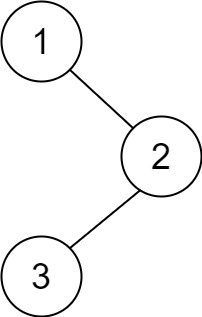``` Input: root = [1,null,2,3] Output: [3,2,1] ```

Example 2:

``` Input: root = [] Output: [] ```

Example 3:

``` Input: root =  Output:  ```

Example 4: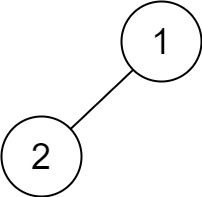``` Input: root = [1,2] Output: [2,1] ```

Example 5: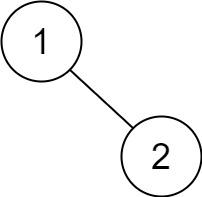``` Input: root = [1,null,2] Output: [2,1] ```

2. Binary Tree Traversal – Solution

The following is a solution which demonstrates how to traverse a binary tree post order.

``` 2. Binary Tree Traversal - Solution C# // ============================================================================ // Author: Kenneth Perkins // Date: Oct 13, 2021 // Taken From: http://programmingnotes.org/ // File: Solution.cs // Description: Demonstrates how to traverse a binary tree post order // ============================================================================ /** * Definition for a binary tree node. * public class TreeNode { * public int val; * public TreeNode left; * public TreeNode right; * public TreeNode(int val=0, TreeNode left=null, TreeNode right=null) { * this.val = val; * this.left = left; * this.right = right; * } * } */ public class Solution { private List<int> result = new List<int>(); public IList<int> PostorderTraversal(TreeNode root) { Traverse(root); return result; } public void Traverse(TreeNode node) { if (node == null) { return; } Traverse(node.left); Traverse(node.right); result.Add(node.val); } }// http://programmingnotes.org/ 12345678910111213141516171819202122232425262728293031323334353637383940 // ============================================================================//    Author: Kenneth Perkins//    Date:   Oct 13, 2021//    Taken From: http://programmingnotes.org///    File:  Solution.cs//    Description: Demonstrates how to traverse a binary tree post order// ============================================================================/** * Definition for a binary tree node. * public class TreeNode { *     public int val; *     public TreeNode left; *     public TreeNode right; *     public TreeNode(int val=0, TreeNode left=null, TreeNode right=null) { *         this.val = val; *         this.left = left; *         this.right = right; *     } * } */public class Solution {    private List<int> result = new List<int>();        public IList<int> PostorderTraversal(TreeNode root) {        Traverse(root);        return result;            }        public void Traverse(TreeNode node) {        if (node == null) {            return;        }                        Traverse(node.left);                Traverse(node.right);                result.Add(node.val);            }    }// http://programmingnotes.org/ ```

QUICK NOTES:
The highlighted lines are sections of interest to look out for.

The code is heavily commented, so no further insight is necessary. If you have any questions, feel free to leave a comment below.

Once compiled, you should get this as your output for the example cases:

``` [3,2,1] []  [2,1] [2,1] ```

## C# || How To Traverse A Binary Tree Preorder Using C#The following is a module with functions which demonstrates how to traverse a binary tree pre order using C#.

1. Binary Tree Traversal – Problem Statement

Given the root of a binary tree, return the preorder traversal of its nodes’ values.

Example 1:``` Input: root = [1,null,2,3] Output: [1,2,3] ```

Example 2:

``` Input: root = [] Output: [] ```

Example 3:

``` Input: root =  Output:  ```

Example 4: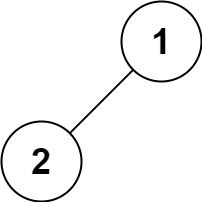``` Input: root = [1,2] Output: [1,2] ```

Example 5:``` Input: root = [1,null,2] Output: [1,2] ```

2. Binary Tree Traversal – Solution

The following is a solution which demonstrates how to traverse a binary tree pre order.

``` 2. Binary Tree Traversal - Solution C# // ============================================================================ // Author: Kenneth Perkins // Date: Oct 13, 2021 // Taken From: http://programmingnotes.org/ // File: Solution.cs // Description: Demonstrates how to traverse a binary tree pre order // ============================================================================ /** * Definition for a binary tree node. * public class TreeNode { * public int val; * public TreeNode left; * public TreeNode right; * public TreeNode(int val=0, TreeNode left=null, TreeNode right=null) { * this.val = val; * this.left = left; * this.right = right; * } * } */ public class Solution { private List<int> result = new List<int>(); public IList<int> PreorderTraversal(TreeNode root) { Traverse(root); return result; } public void Traverse(TreeNode node) { if (node == null) { return; } result.Add(node.val); Traverse(node.left); Traverse(node.right); } }// http://programmingnotes.org/ 12345678910111213141516171819202122232425262728293031323334353637383940 // ============================================================================//    Author: Kenneth Perkins//    Date:   Oct 13, 2021//    Taken From: http://programmingnotes.org///    File:  Solution.cs//    Description: Demonstrates how to traverse a binary tree pre order// ============================================================================/** * Definition for a binary tree node. * public class TreeNode { *     public int val; *     public TreeNode left; *     public TreeNode right; *     public TreeNode(int val=0, TreeNode left=null, TreeNode right=null) { *         this.val = val; *         this.left = left; *         this.right = right; *     } * } */public class Solution {    private List<int> result = new List<int>();        public IList<int> PreorderTraversal(TreeNode root) {        Traverse(root);        return result;            }        public void Traverse(TreeNode node) {        if (node == null) {            return;        }                result.Add(node.val);                Traverse(node.left);                Traverse(node.right);    }}// http://programmingnotes.org/ ```

QUICK NOTES:
The highlighted lines are sections of interest to look out for.

The code is heavily commented, so no further insight is necessary. If you have any questions, feel free to leave a comment below.

Once compiled, you should get this as your output for the example cases:

``` [1,2,3] []  [1,2] [1,2] ```

## C# || How To Traverse A Binary Tree Inorder Using C#The following is a module with functions which demonstrates how to traverse a binary tree in order using C#.

1. Binary Tree Traversal – Problem Statement

Given the root of a binary tree, return the inorder traversal of its nodes’ values.

Example 1:``` Input: root = [1,null,2,3] Output: [1,3,2] ```

Example 2:

``` Input: root = [] Output: [] ```

Example 3:

``` Input: root =  Output:  ```

Example 4:``` Input: root = [1,2] Output: [2,1] ```

Example 5:``` Input: root = [1,null,2] Output: [1,2] ```

2. Binary Tree Traversal – Solution

The following is a solution which demonstrates how to traverse a binary tree in order.

``` 2. Binary Tree Traversal - Solution C# // ============================================================================ // Author: Kenneth Perkins // Date: Oct 13, 2021 // Taken From: http://programmingnotes.org/ // File: Solution.cs // Description: Demonstrates how to traverse a binary tree in order // ============================================================================ /** * Definition for a binary tree node. * public class TreeNode { * public int val; * public TreeNode left; * public TreeNode right; * public TreeNode(int val=0, TreeNode left=null, TreeNode right=null) { * this.val = val; * this.left = left; * this.right = right; * } * } */ public class Solution { private List<int> result = new List<int>(); public IList<int> InorderTraversal(TreeNode root) { Traverse(root); return result; } public void Traverse(TreeNode node) { if (node == null) { return; } Traverse(node.left); result.Add(node.val); Traverse(node.right); } }// http://programmingnotes.org/ 12345678910111213141516171819202122232425262728293031323334353637383940 // ============================================================================//    Author: Kenneth Perkins//    Date:   Oct 13, 2021//    Taken From: http://programmingnotes.org///    File:  Solution.cs//    Description: Demonstrates how to traverse a binary tree in order// ============================================================================/** * Definition for a binary tree node. * public class TreeNode { *     public int val; *     public TreeNode left; *     public TreeNode right; *     public TreeNode(int val=0, TreeNode left=null, TreeNode right=null) { *         this.val = val; *         this.left = left; *         this.right = right; *     } * } */public class Solution {    private List<int> result = new List<int>();     public IList<int> InorderTraversal(TreeNode root) {        Traverse(root);        return result;    }     public void Traverse(TreeNode node) {        if (node == null) {            return;        }         Traverse(node.left);         result.Add(node.val);         Traverse(node.right);    }}// http://programmingnotes.org/ ```

QUICK NOTES:
The highlighted lines are sections of interest to look out for.

The code is heavily commented, so no further insight is necessary. If you have any questions, feel free to leave a comment below.

Once compiled, you should get this as your output for the example cases:

``` [1,3,2] []  [2,1] [1,2] ```